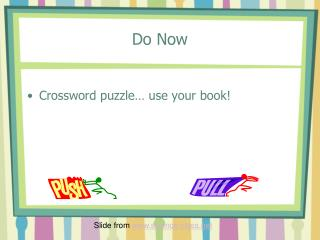DownloadDownload PresentationDo Now

# Do Now

Télécharger la présentation## Do Now

- - - - - - - - - - - - - - - - - - - - - - - - - - - E N D - - - - - - - - - - - - - - - - - - - - - - - - - - -
##### Presentation Transcript

1. Do Now • Crossword puzzle… use your book! Slide from www.science-class.net

2. Newton’s First Law of MotionUnit 2 Day 2

3. First we need to define the word FORCE: • The cause of motion (what causes objects to move) • Two types of forces • Pushes • Pulls Slide from www.science-class.net

4. Forces may be balanced or unbalanced • Balanced forces – all forces acting on an object are equal • There is NO MOTION • NET FORCE = 0 • Unbalanced forces – one or more forces acting on an object are stronger than others • There is MOTION • A NET FORCE

5. Balanced Forces • Forces are balanced when there are equal forces acting on opposite sides of the object. 25N 25N

6. Unbalanced Forces • Forces are unbalanced when there are unequal forces acting on opposite sides of the object. •  25N35N  • Who will win this tug of war??

7. Practice… • If the arrows are pointing in opposite directions… then you subtract the numbers. • 34N 27N

8. Practice… • If the arrows are pointing in the same direction… then you add the numbers. 16N  20N 

9. Practice… • What is the NET FORCE of the two objects below? 12N 32N

10. Objects at Rest • Objects at rest tend to stay at rest unless acted upon by a force. [push or pull] • Newton described this tendency as inertia. • Inertia can be described as the tendency of an object to keep doing whatever’s it’s doing.

11. Mass & Inertia • Mass is the amount of matter in an object. • The more MASS an object has, the more INERTIA the object has. • Bigger objects are harder to start & stop Which vehicle has more inertia? Slide from www.science-class.net

12. What about objects that are already in motion? • Newton stated that objects in motion tend to stay in motion until acted upon by a force (or hits it.)

13. Newton’s 1st Law(also known as the law of inertia) • A moving object moves in a straight line with constant speed unless a force acts on it. • The tendency of an object at rest to remain at rest and an object in motion to remain in motion unless acted upon by an unbalanced force. • Objects do not change their motion unless a force acts on them

14. The truck is in motion. What is the force that causes it to stop? The push of the stopped car. The car is at rest. What is the force that causes it to move? The push of the truck. Slide from www.science-class.net

15. The car is in motion. What is the force that causes it to stop? The push of the stopped car. The wall is at rest. What is the force that causes it to move? The push of the truck. Slide from www.science-class.net

16. Practice… • Now that you understand Newton’s First Law, explain how a car moves and takes you places.

17. Practice… • Now that you understand Newton’s First Law, explain how the space shuttle goes into outer space.

18. Weight vs. Mass • Weight and Mass are NOT the same thing! • Mass – the amount of matter an object contains; the amount of space and object occupies • Weight – the force of gravity on an object

19. Weight vs. Mass • The force of Earth’s gravity is 9.8 N. You can determine the weight of an object using the formula below: W = m x g Weight = mass x gravity

20. Practice… • A sack of groceries has a mass of 10 kg, what is it’s weight. • W = m x g

21. Practice… • A sack of groceries has a mass of 10 kg, what is it’s weight. • W = m x g W = 10 x 9.8 W = 98 N

22. Practice… • Complete questions #1 – 20 on page 56 in your text book… continue on your notes.